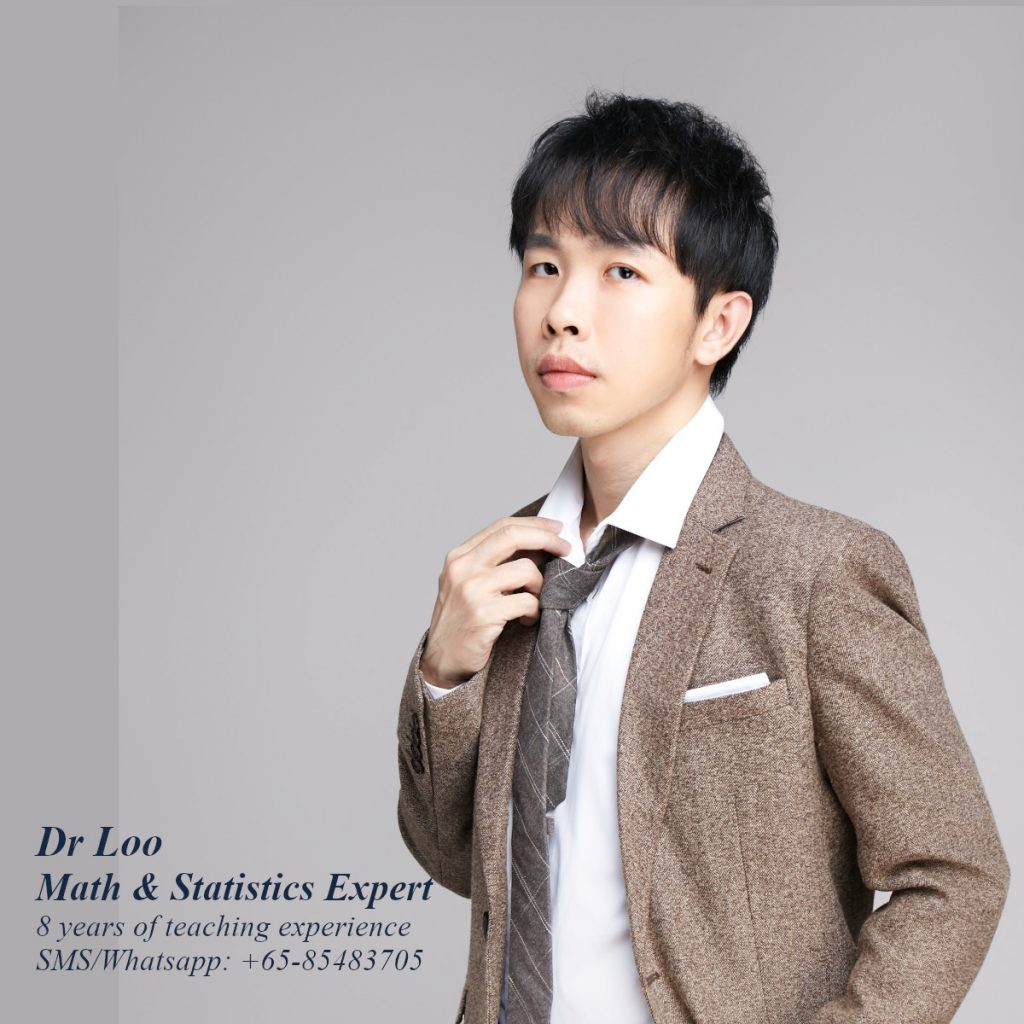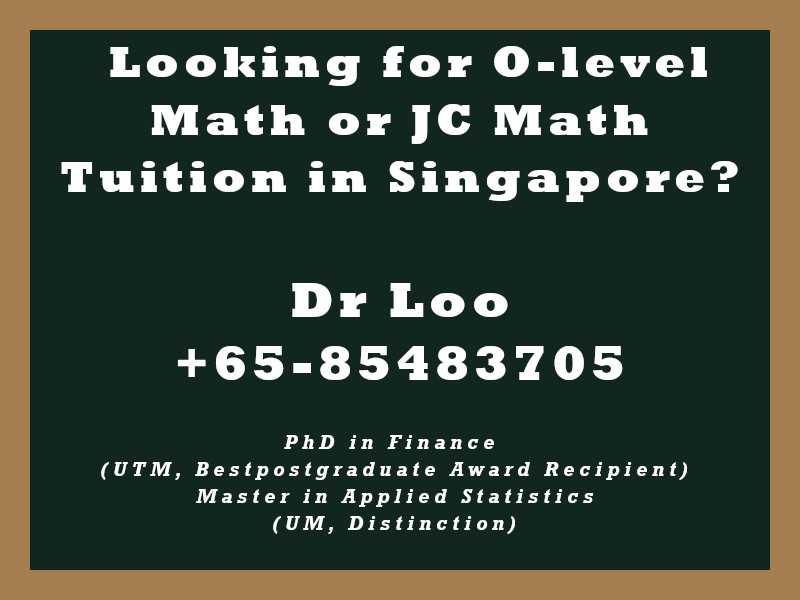+65-85483705
Select Page

# Inverse Function

Question

For each of the functions, obtain its inverse function.

1. g(x) = 2/x
2. g(x) = x + 1
3. g(x) = 3/(x-1)

1. g-1(x) = 2/x
2. g-1(x) = x-1
3. g-1(x) = (3/x) + 1

Note:

• Function f is said to be the inverse of function g if the inverse of function f is also equal to function g and vice versa.
• In order to obtain the inverse function, first one need to set the function x as y then make y become the subject of the formula.
• For verification purpose, one can check if ff-1(x) or f-1f(x) will return to x.

## About the Math Tutor - Dr Loo

I am a PhD holder with 9 years of teaching experience at secondary school and university. My expertizes are mathematics, statistics, econometrics, finance and machine learning.

Currently I do provide consultation and private tuition in Singapore. For those who are looking for math tutor in Singapore or statistics tutor in Singapore, please feel free to contact me at +65-85483705 (SMS/Whatsapp/Telegram).

Some of the math tuition in Singapore I provide includes O-level math tuition and also JC Math Tuition (H1 Math Tuition & H2 Math Tuition). On the other hand, my statistics tuition in Singapore mostly focus on pre-university level until postgraduate level. For those who prefer online tutoring service, the online O-level math tuition and online JC math tuition are also available.## For those who are looking for math tution in Singapore

Need help with this topic? I do provide mathematics home tuition in Singapore for O-level math and also JC H2 math. In addition, online math tutoring is available as well. Feel free to contact me if you would like to know further.